Home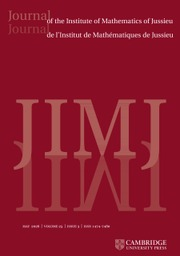Journal of the Institute of Mathematics of Jussieu

# EPSTEIN ZETA-FUNCTIONS, SUBCONVEXITY, AND THE PURITY CONJECTURE

Published online by Cambridge University Press:  02 April 2018

Corresponding

## Abstract

Subconvexity bounds on the critical line are proved for general Epstein zeta-functions of$k$ -ary quadratic forms. This is related to sup-norm bounds for unitary Eisenstein series on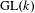$\text{GL}(k)$ associated with the maximal parabolic of type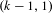$(k-1,1)$ , and the exact sup-norm exponent is determined to be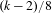$(k-2)/8$ for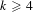$k\geqslant 4$ . In particular, if$k$ is odd, this exponent is not in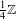$\frac{1}{4}\mathbb{Z}$ , which is relevant in the context of Sarnak’s purity conjecture and shows that it can in general not directly be generalized to Eisenstein series.

Type
Research Article
Information

## Access options

Get access to the full version of this content by using one of the access options below.

## Footnotes

The author was supported in part by SNF-DFG grant BL 915/2 and NSF grant 1128155 while enjoying the hospitality of the Institute for Advanced Study. The United States Government is authorized to reproduce and distribute reprints notwithstanding any copyright notation herein.

## References

Andersson, J. and Södergren, A., On the universality of the Epstein zeta function, Preprint, 2015, arXiv:1508.05836.Google Scholar
Arthur, J., A trace formula for reductive groups. II. Applications of a truncation operator, Compos. Math. 40 (1980), 87121.Google Scholar
Balasubramanian, R., Ivić, A. and Ramachandra, K., The mean square of the Riemann zeta-function on the line 𝜎 = 1, Enseign. Math. 38 (1992), 1325.Google Scholar
Blomer, V., Subconvexity for a double Dirichlet series, Compos. Math. 147 (2011), 355374.CrossRefGoogle Scholar
Blomer, V., Khan, R. and Young, M., Distribution of mass of holomorphic cusp forms, Duke Math. J. 162 (2013), 26092644.CrossRefGoogle Scholar
Brumley, F. and Templier, N., Large values of cusp forms on$\text{GL}(n)$, Preprint, 2013,arXiv:1411.4317.Google Scholar
Chandrasekharan, K. and Narasimhan, R., The approximate functional equation for a class of zeta-functions, Math. Ann. 152 (1963), 3064.CrossRefGoogle Scholar
Cohen, H., Sums involving the values at negative integers of L-functions of quadratic characters, Math. Ann. 217 (1975), 271285.CrossRefGoogle Scholar
Donnelly, H., Exceptional sequences of eigenfunctions for hyperbolic manifolds, Proc. Amer. Math. Soc. 135 (2007), 15511555.CrossRefGoogle Scholar
Epstein, P., Zur Theorie allgemeiner Zetafunctionen, Math. Ann. 56 (1903), 615644.CrossRefGoogle Scholar
Fomenko, O. M., The order of the Epstein zeta-function in the critical strip, J. Math. Sci. (N.Y.) 110 (2002), 31503163. translation.CrossRefGoogle Scholar
Goldfeld, D., Automorphic forms and L-functions for the group GL(n, ℝ), Cambridge Studies in Advanced Mathematics, Volume 99 (Cambridge University Press, 2006).Google Scholar
Good, A., Beiträge zur Theorie der Dirichletreihen, die Spitzenformen zugeordnet sind, J. Number Theory 13 (1981), 1865.CrossRefGoogle Scholar
Götze, F., Lattice point problems and values of quadratic forms, Invent. Math. 157 (2004), 195226.CrossRefGoogle Scholar
Hecke, E., Über Modulfunktionen und Dirichletsche Reihen mit Eulerscher Produktentwicklung II, Math. Ann. 114 (1937), 316351.CrossRefGoogle Scholar
Iwaniec, H. and Kowalski, E., Analytic Number Theory, Volume 53 (AMS Colloquium Publications, Providence, 2004).Google Scholar
Iwaniec, H. and Sarnak, P., L norms of eigenfunctions of arithmetic surfaces, Ann. of Math. 141 (1995), 301320.CrossRefGoogle Scholar
Iwaniec, H. and Sarnak, P., Perspectives on the analytic theory of L-functions, GAFA Special volume, pp. 705741. (2000).Google Scholar
Jarník, V., Über Gitterpunkte in mehrdimensionalen Ellipsoiden, Math. Ann. 100 (1928), 699721.CrossRefGoogle Scholar
Lapid, E. and Offen, O., Compact unitary periods, Compositio Math. 143 (2007), 323338.CrossRefGoogle Scholar
Milićević, D., Large values of eigenfunctions on arithmetic hyperbolic surfaces, Geom. Funct. Anal. 21 (2011), 13751418.CrossRefGoogle Scholar
Miller, S. D., On the existence and temperedness of cusp forms for SL3(ℤ), J. Reine Angew. Math. 533 (2001), 127169.Google Scholar
Rudnick, Z. and Sarnak, P., The behavior of eigenstates of arithmetic hyperbolic manifolds, Comm. Math. Phys. 161 (1994), 195213.CrossRefGoogle Scholar
Sarnak, P., Letter to Morawetz http://www.math.princeton.edu/sarnak.Google Scholar
Shimura, G., The Representation of Integers as Sums of Squares, Amer. J. Math. 124 (2002), 10591081.CrossRefGoogle Scholar
Siegel, C. L., Über die analytische Theorie der quadratischen Formen, Ann. of Math. 36 (1935), 527606.CrossRefGoogle Scholar
Sono, K., Higher moments of the Epstein zeta functions, Tokyo J. of Math. 36 (2013), 269287.CrossRefGoogle Scholar
Spinu, F., The$L^{4}$ norm of the Eisenstein series, thesis, Princeton (2003).Google Scholar
Titchmarsh, E. C., On Epstein’s zeta-function, Proc. Lond. Math. Soc. (2) 36 (1934), 485500.CrossRefGoogle Scholar
Young, M., A note on the sup norm of Eisenstein series, Quart. J. Math., to appear.Google Scholar
Zhang, L., Quantum unique ergodicity of degenerate Eisenstein series on$\text{GL}(n)$, Preprint, 2016, arXiv:1609:01386.Google Scholar

### Full text views

Full text views reflects PDF downloads, PDFs sent to Google Drive, Dropbox and Kindle and HTML full text views.

Total number of HTML views: 0
Total number of PDF views: 144 *
View data table for this chart

* Views captured on Cambridge Core between 02nd April 2018 - 25th January 2021. This data will be updated every 24 hours.

Hostname: page-component-76cb886bbf-2rmft Total loading time: 0.23 Render date: 2021-01-25T02:26:18.222Z Query parameters: { "hasAccess": "0", "openAccess": "0", "isLogged": "0", "lang": "en" } Feature Flags: { "shouldUseShareProductTool": true, "shouldUseHypothesis": true, "isUnsiloEnabled": true, "metricsAbstractViews": false, "figures": false, "newCiteModal": false }

# Send article to Kindle

Note you can select to send to either the @free.kindle.com or @kindle.com variations. ‘@free.kindle.com’ emails are free but can only be sent to your device when it is connected to wi-fi. ‘@kindle.com’ emails can be delivered even when you are not connected to wi-fi, but note that service fees apply.

Find out more about the Kindle Personal Document Service.

EPSTEIN ZETA-FUNCTIONS, SUBCONVEXITY, AND THE PURITY CONJECTURE
Available formats
×

# Send article to Dropbox

To send this article to your Dropbox account, please select one or more formats and confirm that you agree to abide by our usage policies. If this is the first time you use this feature, you will be asked to authorise Cambridge Core to connect with your <service> account. Find out more about sending content to Dropbox.

EPSTEIN ZETA-FUNCTIONS, SUBCONVEXITY, AND THE PURITY CONJECTURE
Available formats
×

# Send article to Google Drive

To send this article to your Google Drive account, please select one or more formats and confirm that you agree to abide by our usage policies. If this is the first time you use this feature, you will be asked to authorise Cambridge Core to connect with your <service> account. Find out more about sending content to Google Drive.

EPSTEIN ZETA-FUNCTIONS, SUBCONVEXITY, AND THE PURITY CONJECTURE
Available formats
×
×